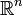# 1617-257/TUT-R-3

On 9/29/16, we discussed three notions of compactness in$\mathbb{R}^n$ equipped with the usual topology:

(1) closed and bounded

(2) subsequential compactness

(3) every open cover admits a finite subcover

We will tacitly assume that this is the topology we're giving$\mathbb{R}^n$ for the remainder of this post.

• We proved that (1) and (2) are equivalent.
• Statements (2) and (3) are equivalent in general metric spaces.
• (1) is not necessarily equivalent to (2) or (3) in other settings (and even non-contrived settings: that is, settings which are not just around for the sake of counterexample. There is an abundance of examples arising from basic objects of study in functional analysis.).